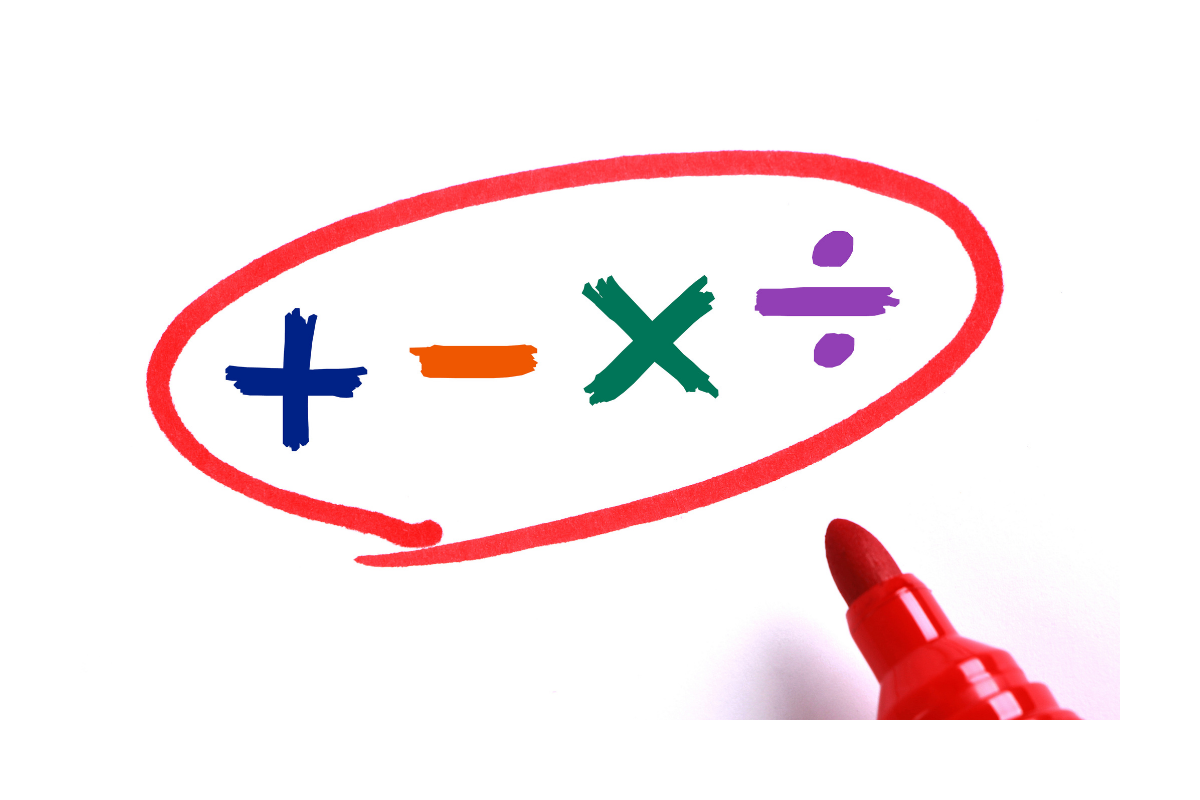Picture This! - Exceed in Learning

# Picture This!As adults, we understand the importance of drawing a visual to help us understand complex ideas.  The same idea is used by teachers, elementary through high school.

Many teachers/parents continue to use drawings with their students/children to enhance their math instruction.  Our students and children can get excited about math with pictures and visuals.  We can use this to show children that math isn’t just about formulas, shortcuts, etc.  It is about recognizing and understanding patterns and then applying what we know to what we see.

A strategy that many primary teachers use when teaching math is the CRT method.  This stands for concrete, representational, abstract.  Think back to when children are first learning how to add.  A child may first use unifix cubes to represent the problem 5 + 2.  This is something concrete that they can put their hands on.  Once they understand how to use the cubes to add, they move to drawings to take the place of the physical cubes.  One the student understands how to solve the problem with drawings, they move to the “abstract” phase.  This is what most people know math as – just the numbers.  Many times, we try to teach a concept so quickly that we forget the concrete and representational stages.  We move straight to the abstract phase.  For young students, it is imperative that they are given ample time to practice a new concept with both concrete and representational activities.

As students get into more advanced math, the concrete phase begins to fade out, but there are still many opportunities for students to “draw it out”.  This particularly is used when working with word problems.  Word problems can be difficult, and teachers have used drawings for a long time.  To help children make sense of word problems, the pictures will make it much more understandable for them.  Let’s take a look at how pictures may help with different math topics:

Counting, addition, and subtraction are the main focus of primary-age children.  Consider finding some pictures where there are many items.  For example, challenge the child to count the number of lions and the number of tigers.  They can then work to find the total number of animals.  Students can also work on counting by 2s, finding missing numbers, etc.

Pictures with multiplication and division are typically done by means of arrays and groups.  These activities are very fun to do with small candies, such as M&Ms or skittles.  You can have your child place the candies in equal rows to show a multiplication problem.  What a fun snack-tivity!

Teaching fractions can be a bit tricky.  Legos can be a great way to show the difference between different fraction benchmarks (1/2/, 1/3, ¼).  Additionally, cooking with measuring cups is a great way to practice comparing fractions, adding fractions, etc.

What are some of the ways you use visuals and drawings to teach math?